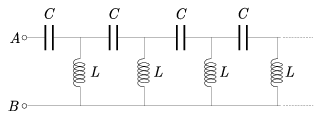Mathematical and Physical Journal
for High Schools
Issued by the MATFUND Foundation
 Already signed up? New to KöMaL?

# Exercises and problems in PhysicsApril 2002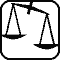## Experimental problem

M. 233. Put a small sized glass filled with water on a matchbox lying on a table. Use a ruler to knock the matchbox out from under the glass. Take simple measurements (for example among others measure the displacement of the glass), and with the help of these estimate how much time it took to knock the matchbox out. (6 points)## Theoretical problems

P. 3520. Two trains are running along two parallel tracks. One of them covers 90 km an hour the other 10 meters a second. A passenger sitting in one of the trains observes that the other train passes in 8 seconds. How long is the other train? (3 points)

P. 3521. Pour 10 cm3 of water into an empty cube with 1,01 dm long edges, and then put an oak cube of a mass of 0,7 kg into it. Does the oak cube float? (4 points)

P. 3522. There is a 0,8 m long homogeneous stick attached with a hinge to the rim of a big tank partially filled with water. The density of the material of the stick is 5 kg/dm3. If a 650 cm3 sphere of density of 0,4 kg/dm3 is fixed at the other end of the stick, then the sphere submerges in the water and so does three quarters of the stick.

a) What is the cross section of the stick.

b) How much is the force and what is its direction exerted by the hinge on the stick? (4 points)

P. 3523. The figure (see on page 251) shows the velocity and the acceleration of a point-like body at the initial moment of its motion. The direction and the absolute value of the acceleration remain constant. a) When does the absolute value of the velocity become the same as at the initial moment?

b) When does the velocity reach its minimum value?

c) What is the minimal curvature of the orbit of the body?

(Data: a=6 m/s2, v0=24 m/s, $\displaystyle varphi$=120o.) (5 points)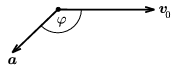P. 3524. The medium (ammonia, freon) in a compression refrigerator and heater (heat pump) goes through the same thermodynamic process in each cycle. What does it depend on whether it works as a refrigerator or a heater? (4 points)

P. 3525. There is a d distance between the big sized plates of a plate capacitor charged up to voltage U. From the negative plate a particle of charge Q and mass m is detached without an initial velocity. At what velocity will this negative charge hit the positive plate? What is the U voltage where this velocity is minimal? (5 points)

P. 3526. At the two ends of an electric insulator pipe the ends of which are bent at right angles there are soap bubbles of radius r and R, respectively. How much electric charge is to be added to one of the bubbles to ensure that the size of the other bubble does not change after opening the tap at the middle of the pipe? (Neglect the effect of the electrostatic induction!) (5 points)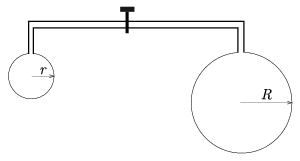P. 3527. A magnetic rod is dropped into a very long vertical short-circuited coil as seen in the figure. Make a schematic drawing of the currents in the coil and the acceleration of the magnetic rod as a function of time.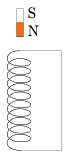(5 points)

P. 3528. The boundary surface of the media of refractive indices n1 and n2 in the optical system that can be seen in the figure is a sphere of radius R. Is there a point on axis t where light beams starting from (closing only a small angle with axis t) are running parallel to axis t in the other medium? (5 points)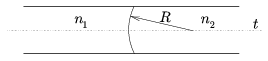P. 3529. At the beginning of the 20th century the Sun was considered a 6000 K hot glowing homogeneous gas sphere consisting of H2 molecules, and slowly cooling down by its radiation. The current intensity of the Sun's radiation at the distance of the Earth is 1400 W/m2. Estimate how long it would take for the Sun to darken, that is to cool down to about 1000 K? (According to our present knowledge, the Sun - similarly to other stars - maintains its radiation from the energy released in fusion processes of atomic nuclei and will probably shine for about 5 billion years from now on.) (5 points)

P. 3530. What is the apparent resistance of the endless chain seen in the figure in alternating voltage at circular frequency (between terminals A and B? Can the result be two different values? (6 points)